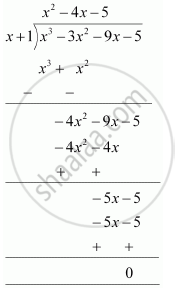# Factorise :- x^3 – 3x^2 – 9x – 5 - Mathematics

Factorise :- x3 – 3x2 – 9x – 5

#### Solution

Let p(x) = x3 − 3x2 − 9− 5

All the factors of 5 have to be considered. These are ±1, ± 5.

By trial method,

p(−1) = (−1)3 − 3(−1)2 − 9(−1) − 5

= − 1 − 3 + 9 − 5

= 0

Therefore, x + 1 is a factor of this polynomial.

Let us find the quotient on dividing x3 + 3x2 − 9− 5 by x + 1.

By long division,It is known that,

Dividend = Divisor × Quotient + Remainder

∴ x3 − 3x2 − 9− 5 = (+ 1) (x2 − 4x − 5) + 0

= (+ 1) (x2 − 5x + x − 5)

= (x + 1) [((x − 5) +1 (x − 5)]

= (x + 1) (x − 5) (x + 1)

= (x − 5) (x + 1) (x + 1)

Concept: Factorising the Quadratic Polynomial (Trinomial) of the type ax2 + bx + c, a ≠ 0.
Is there an error in this question or solution?
Chapter 2: Polynomials - Exercise 2.4 [Page 44]

#### APPEARS IN

NCERT Class 9 Maths
Chapter 2 Polynomials
Exercise 2.4 | Q 5.2 | Page 44

Share